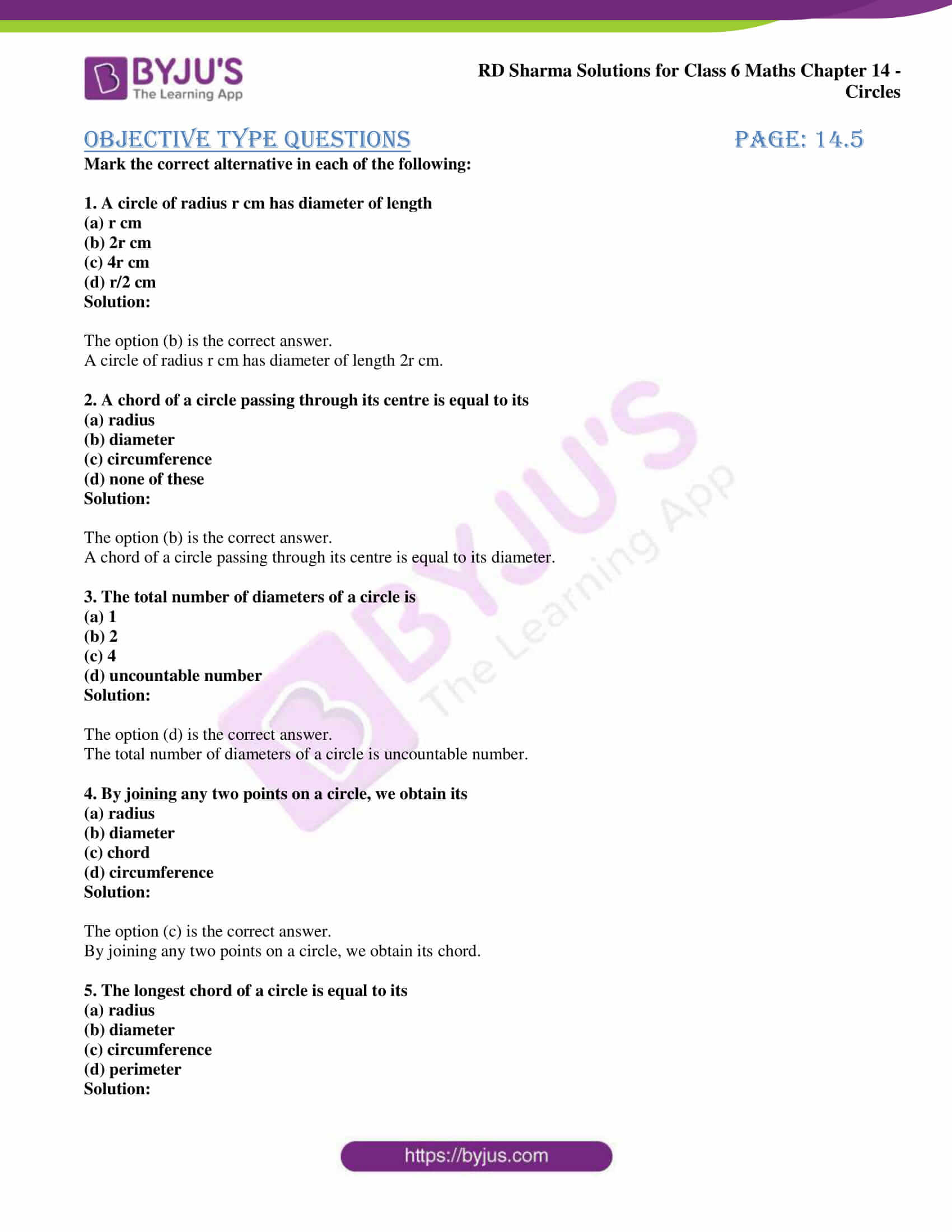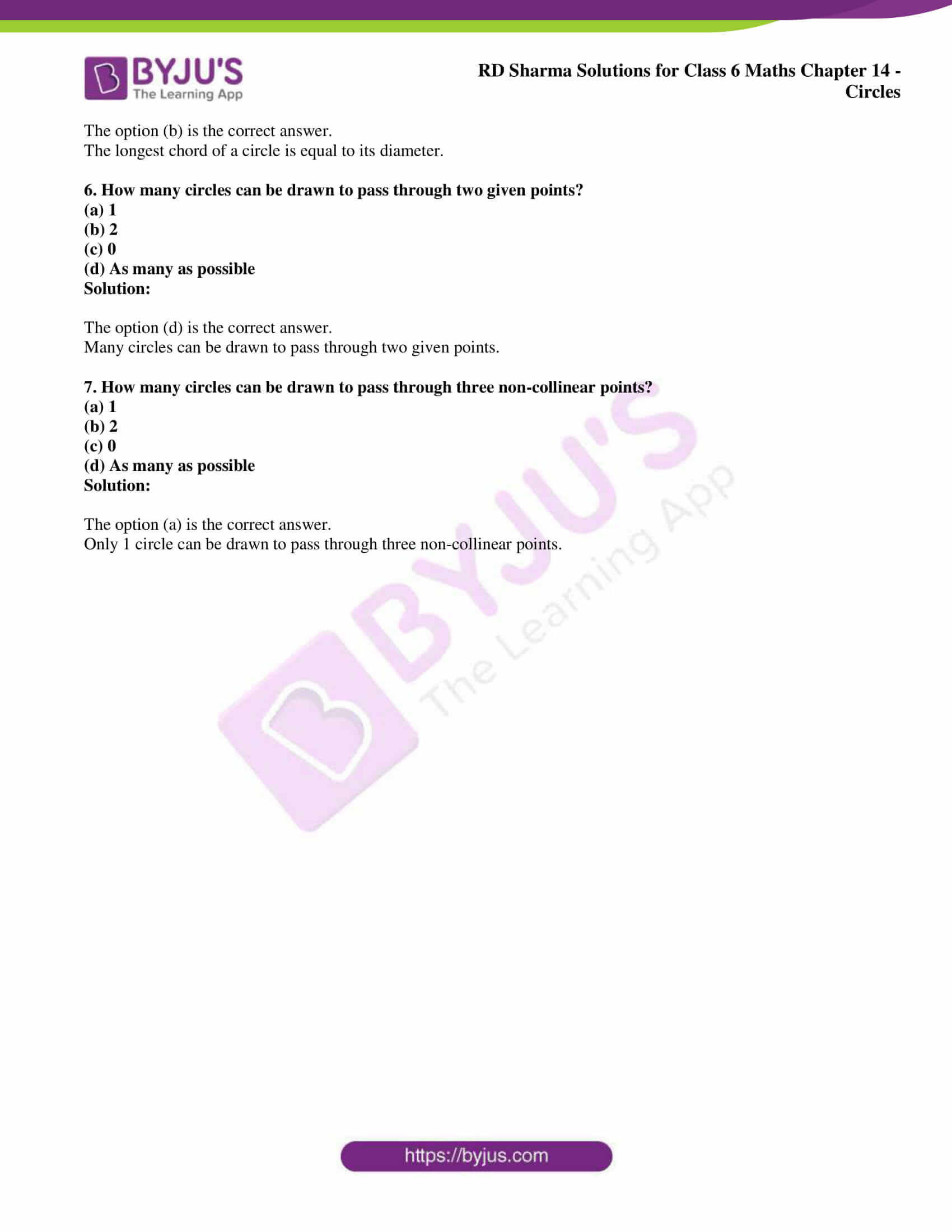# RD Sharma Solutions for Class 6 Maths Chapter 14: Circles Objective Type Questions

This Chapter has objective type questions at the end in order to help students analyse their overall knowledge about the concepts. These solutions are prepared in short using important formulas and tricks to make them simple for the students during exam preparation. The primary objective of providing exercise wise solutions to the students is to help them perform well in the exam. RD Sharma Solutions Class 6 Maths Chapter 14 Circles Objective Type Questions PDF can be downloaded from the links which are provided here.

## RD Sharma Solutions for Class 6 Maths Chapter 14: Circles Objective Type Questions Download PDF### Objective Type Questions page: 14.5

Mark the correct alternative in each of the following:

1. A circle of radius r cm has diameter of length
(a) r cm
(b) 2r cm
(c) 4r cm
(d) r/2 cm

Solution:

The option (b) is the correct answer.

A circle of radius r cm has diameter of length 2r cm.

2. A chord of a circle passing through its centre is equal to its
(b) diameter
(c) circumference
(d) none of these

Solution:

The option (b) is the correct answer.

A chord of a circle passing through its centre is equal to its diameter.

3. The total number of diameters of a circle is
(a) 1
(b) 2
(c) 4
(d) uncountable number

Solution:

The option (d) is the correct answer.

The total number of diameters of a circle is uncountable number.

4. By joining any two points on a circle, we obtain its
(b) diameter
(c) chord
(d) circumference

Solution:

The option (c) is the correct answer.

By joining any two points on a circle, we obtain its chord.

5. The longest chord of a circle is equal to its
(b) diameter
(c) circumference
(d) perimeter

Solution:

The option (b) is the correct answer.

The longest chord of a circle is equal to its diameter.

6. How many circles can be drawn to pass through two given points?
(a) 1
(b) 2
(c) 0
(d) As many as possible

Solution:

The option (d) is the correct answer.

Many circles can be drawn to pass through two given points.

7. How many circles can be drawn to pass through three non-collinear points?
(a) 1
(b) 2
(c) 0
(d) As many as possible

Solution:

The option (a) is the correct answer.

Only 1 circle can be drawn to pass through three non-collinear points.